# Multiplication of a Vector by a Scalar

Go back to  'Vectors'

Consider a vector $$\vec a$$. What happens if you multiply this vector by 2? What will the vector $$2\vec a$$ represent?

Intuitively, the answer should be clear: the new vector will be twice the length of the original vector, but in the same direction: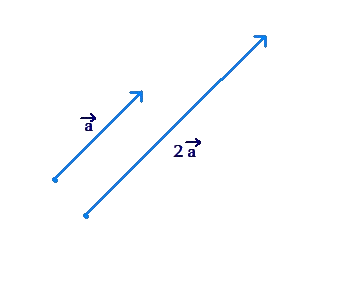The vector $$\frac{1}{2}\vec a$$ will be a vector in the same direction as $$\vec a$$, but with a length equal to half of the length of $$\vec a$$: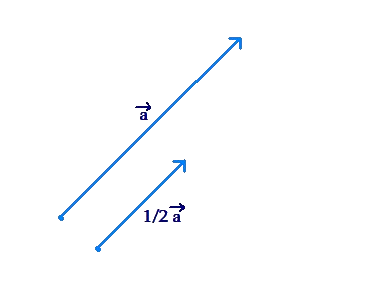We have seen how to interpret the vector $$- \vec a$$, given the vector $$\vec a$$: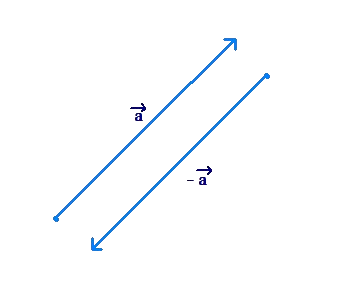The two vectors are in opposite directions.

What about the vector $$- 2\vec a$$? The direction of this vector will be opposite to the direction of $$\vec a$$, and its length will be twice the length of $$\vec a$$: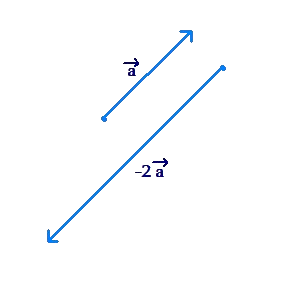The vector $$- \frac{1}{2}\vec a$$ will have a direction opposite to that of $$\vec a$$, and will have a length equal to half the length of $$\vec a$$: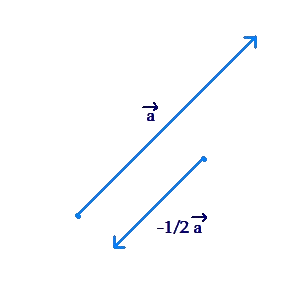To summarize:

• Multiplying a vector by a positive scalar (real number) preserves its direction, and scales its length by the magnitude of the scalar.

• Multiplying a vector by a negative scalar reverses its direction, and scales its length by the magnitude of the scalar.

• It the magnitude of the scalar is greater than 1, then the new vector is longer than the original vector; if it is less than 1, then the new vector is shorter; if the scalar is equal to 1, the new vector has the same length as the original.

Vectors
grade 10 | Questions Set 1
Vectors
Vectors
grade 10 | Questions Set 2
Vectors